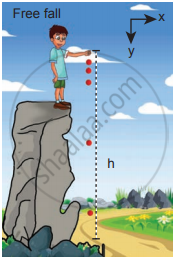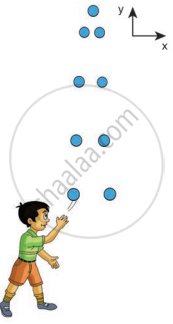Tamil Nadu Board of Secondary EducationHSC Science Class 11th

# Derive the equations of motion for a particle (a) falling vertically (b) projected vertically. - Physics

Derivation

Derive the equations of motion for a particle (a) falling vertically (b) projected vertically.

#### Solution

’Equations of motion for a particle falling vertically downward from a certain height. Consider an object of mass m falling from a height h. Assume there is no air resistance. For convenience, let us choose the downward direction as a positive y-axis as shown in the figure. The object experiences acceleration ‘g’ due to gravity which is constant near the surface of the Earth. We can use kinematic equations to explain its motion. We have The acceleration veca = g hati
By comparing the components, we get,
Equations of motion for a particle thrown vertically upwards,
ax = 0, ax = 0, ay = g Let us take for simplicity, ay = a = gAn object in free fall

If the particle is thrown with initial velocity ‘u’ downward which is in the negative y-axis, then velocity and position at of the particle any time t is given by
v = u + gt
v = ut + 1/2 – gt2
The square of the speed of the particle when it is at a distance y from the hilltop is v2 = u2 + 2 gy
Suppose the particle starts from rest.
Then u = 0
Then the velocity v, the position of the particle, and v2 at any time t are given by (for a point y from the hilltop)
v = gt …………(i)
y = 1/2 – gt2 …………(ii)
v2 = 2gy …………(iii)
The time (t = T) taken by the particle to reach the ground (for which y = h), is given by using equation (ii),
h = 1/2 – gT2 …………(iv)
T = sqrt((2h)/g) ......................(v)

The equation (iv) implies that the greater the height (h), the particle takes more time (T) to reach the ground. For lesser height (h), it takes lesser time to reach the ground. The speed of the particle when it reaches the ground (y = h) can be found using equation (iii), we get,

v_"ground" = sqrt(2gh) ...................(Vi)

The above equation implies that the body falling from a greater height (h) will have higher velocity when it reaches the ground. The motion of a body falling towards the Earth from a small altitude (h<<R), purely under the force of gravity is called free fall. (Here R is the radius of the Earth).

case (ii):
A body is thrown vertically upwards:
Consider an object of mass m thrown vertically upwards with an initial velocity u. Let us neglect the air friction. In this case, we choose the vertical direction as the positive y-axis as shown in the figure, then the acceleration a = -g (neglect air friction) and g points towards the negative y-axis. The kinematic equations for this motion are,
The velocity and position of the object at any time t are,An object is thrown vertically

v = u – gt ……………(vii)
s = ut – 1/2 – gt2 …………..(viii)
The velocity of the object at any position y (from the point where the object is thrown) is
v2 = u2 – 2gy …………..(ix)

Concept: Concept of Rest and Motion
Is there an error in this question or solution?

Share